Question

# To find the mass percent of dolomite, or CaMg(CO3)2, in a soil sample, a geochemist titrates...

To find the mass percent of dolomite, or CaMg(CO3)2, in a soil sample, a geochemist titrates 13.96 g of soil with 40.31 mLof 0.2516 M HCl.

What is the mass percent of dolomite in the soil?

_____% dolomite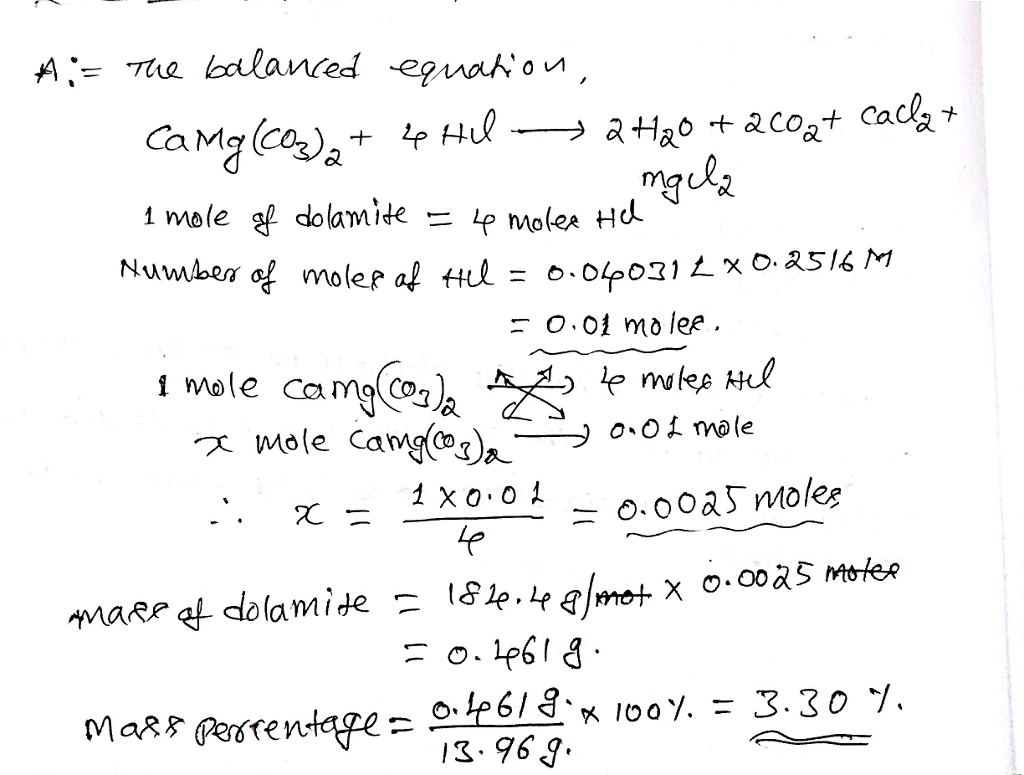#### Earn Coins

Coins can be redeemed for fabulous gifts.

Similar Homework Help Questions
• ### Dolomite, [CaMg(CO3)2] is found in a soil sample. A geochemist titrates 24.65 g of soil with...

Dolomite, [CaMg(CO3)2] is found in a soil sample. A geochemist titrates 24.65 g of soil with 57.85 mL of 0.3315M HCl. What is the mass % of dolomite in the soil?

• ### CE 321 Soil 1. A block sample of soil the soil sample was used for gravity...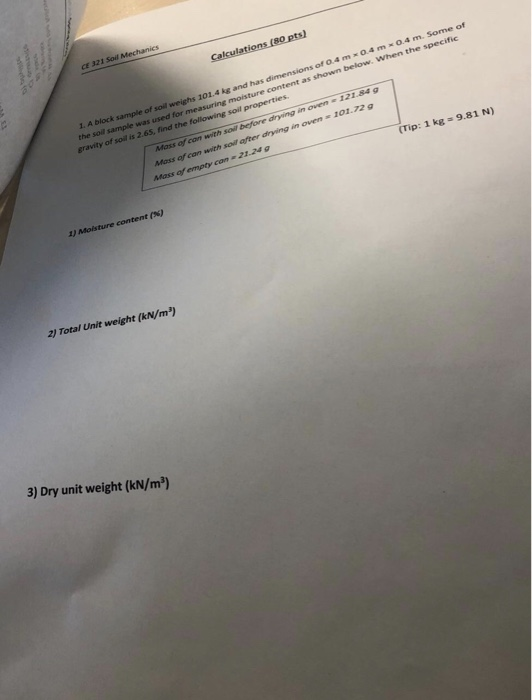CE 321 Soil 1. A block sample of soil the soil sample was used for gravity of soil is 2.65, find the following soil 101.4 kg and has dimensions of 0.4mx0.4 mx0.4 m. Some of moisture content as shown below. When the specific Mass of can with soil before drying in oven1z1.84g Mass of can with soil after drying in oven 101.72 (Tip: 1 kg 9.81 N) content ( 2) Total Unit weight (kN/m3) 3) Dry unit weight (kN/m') CE...

• ### 0.1081 g of an unknown compound containing CO3^2- was added into 50 mL of distilled water....

0.1081 g of an unknown compound containing CO3^2- was added into 50 mL of distilled water. It took 14.05 mL of 0.1 M HCl to reach equivalence point 2 from titration. What is the percent carbonate CO3^-2 of the unknown compound?

• ### undisturbed soil sample has a total mass of 2290 g and volume of 1.15 x 10...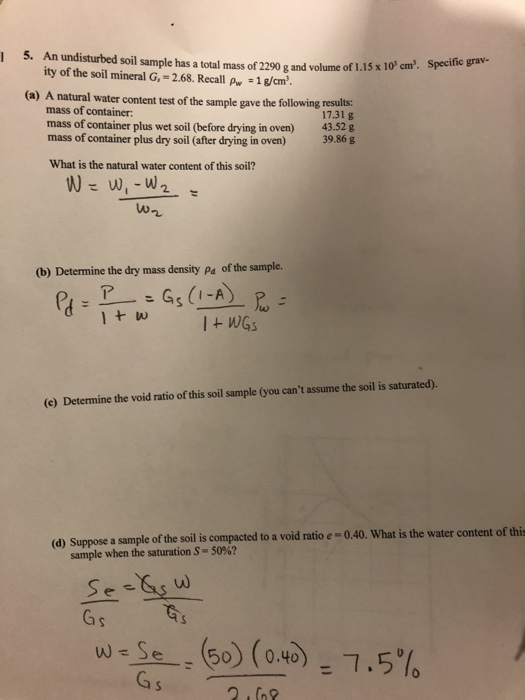undisturbed soil sample has a total mass of 2290 g and volume of 1.15 x 10 cm'. Specifica ity of the soil mineral G. = 2.68. Recall pw = 1 g/cm'. (a) A natural water content test of the sample gave the following results mass of container: 17.31 g mass of container plus wet soil (before drying in oven) 43.52 g mass of container plus dry soil (after drying in oven) 39.86 g What is the natural water content of...

• ### Finding mass percent of a compund in its original sample

A sample of limestone and other soil materials is heated, and the lilmestone decomposes to give calcium oxide and carbon dioxide.CaCO3 (s) = CaO(s) + CO2(g)A 1.506-g sample of limestone-containing material gives .558 g of CO2 in addition ti CaO, after being heated at a high temperature. What is the mass percent of CaCO3in the original sample?

• ### please do problem 3 Problem 1: In its natural condition a soil sample has a mass...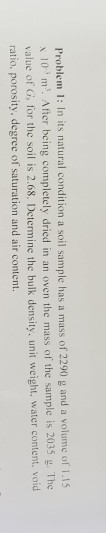please do problem 3 Problem 1: In its natural condition a soil sample has a mass of 2290 g and a volume of 1.15 x10 m. After being completely dried in an oven the mass of the sample is 2035 g. The value of G, for the soil is 2.68. Determine the bulk density, unit weight, water content. void ratio, porosity, degree of saturation and air content. Problem 3: A soil specimen is 38 mm in diameter and 76 mm...

• ### III. Preparing for next week: A student titrates 2.025 g of vinegar with 0.121 M NaOH. It takes 14.01 mL of NaOH to...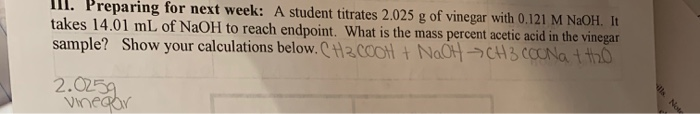III. Preparing for next week: A student titrates 2.025 g of vinegar with 0.121 M NaOH. It takes 14.01 mL of NaOH to reach endpoint. What is the mass percent acetic acid in the vinegar sample? Show your calculations below.CH.COOH + NaOH > CH3COONa the 2.0259 Wechy

• ### 1. A soil sample is taken from a small test hole in a soil borrow pit....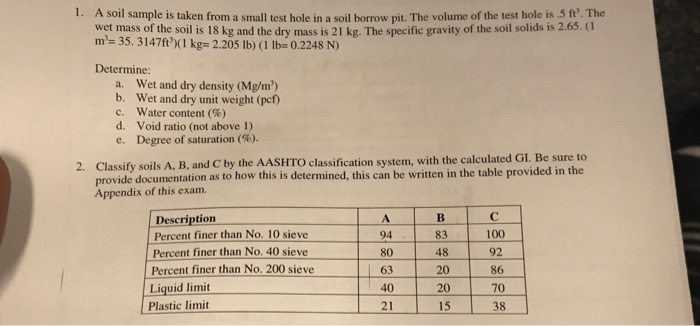1. A soil sample is taken from a small test hole in a soil borrow pit. The volume of the test hole is 5 ft. The wet mass of the soil is 18 kg and the dry mass is 21 kg. The specific gravity of the soil solids is 2.65. ( m 35. 3147ft)(1 kge 2.205 lb) ( 1 lb= 0.2248 N) Determine: a. Wet and dry density (Mg/m) b. Wet and dry unit weight (pcf) C. Water content (%)...

• ### Soil Mechanics (300985) - Practice for final exam SESSION 2 SCC 2018 Question 1 a C...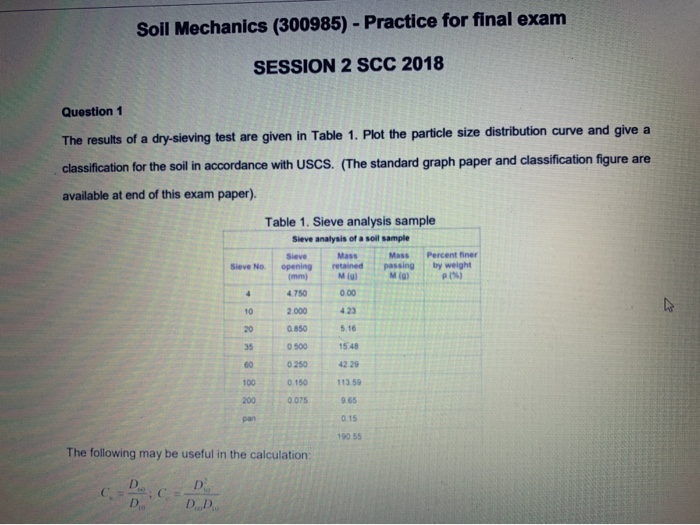Soil Mechanics (300985) - Practice for final exam SESSION 2 SCC 2018 Question 1 a C The results of a dry-sieving test are given in Table 1. Plot the particle size distribution curve and give classification for the soil in accordance with USCS. (The standard graph paper and classification figure are paper). available at end of this exam Table 1. Sieve analysis sample Sieve analysis of a soil sample Mass Percent finer Sieve Mass by weight passing M(g) Sieve No....

• ### A 170 cm sample of soil obtained from below the ground water table has a mass...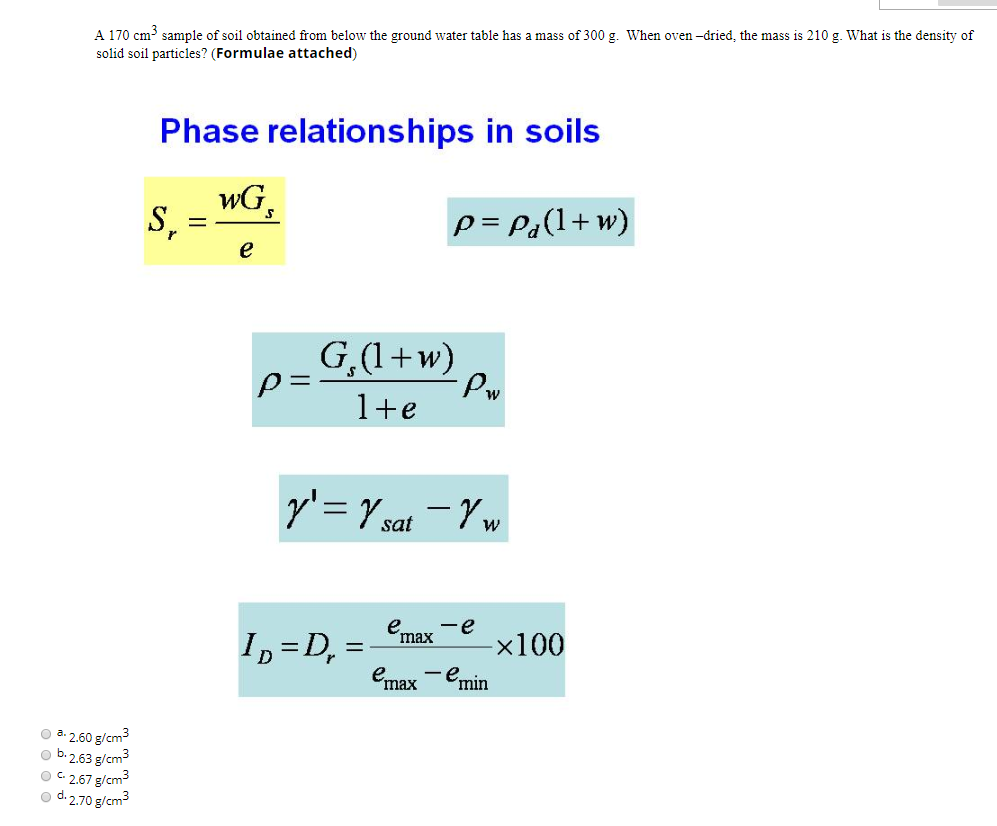A 170 cm sample of soil obtained from below the ground water table has a mass of 300 g. When oven-dried, the mass is 210 g. What is the density of solid soil particles? (Formulae attached) Phase relationships in soils S, = WG p=Pa(1+w) p=GWm y'= 7 sat – Yw 1. =D, = {max e x100 emax - emin a. 2.60 g/cm3 b.2.63 g/cm3 OC.2.67 g/cm3 d. 2.70 g/cm3